Published

# Smartap Future of Faucets

An advanced faucet that can close if left open, can monitor the water usage, can control the water flow to manage water consumption at home.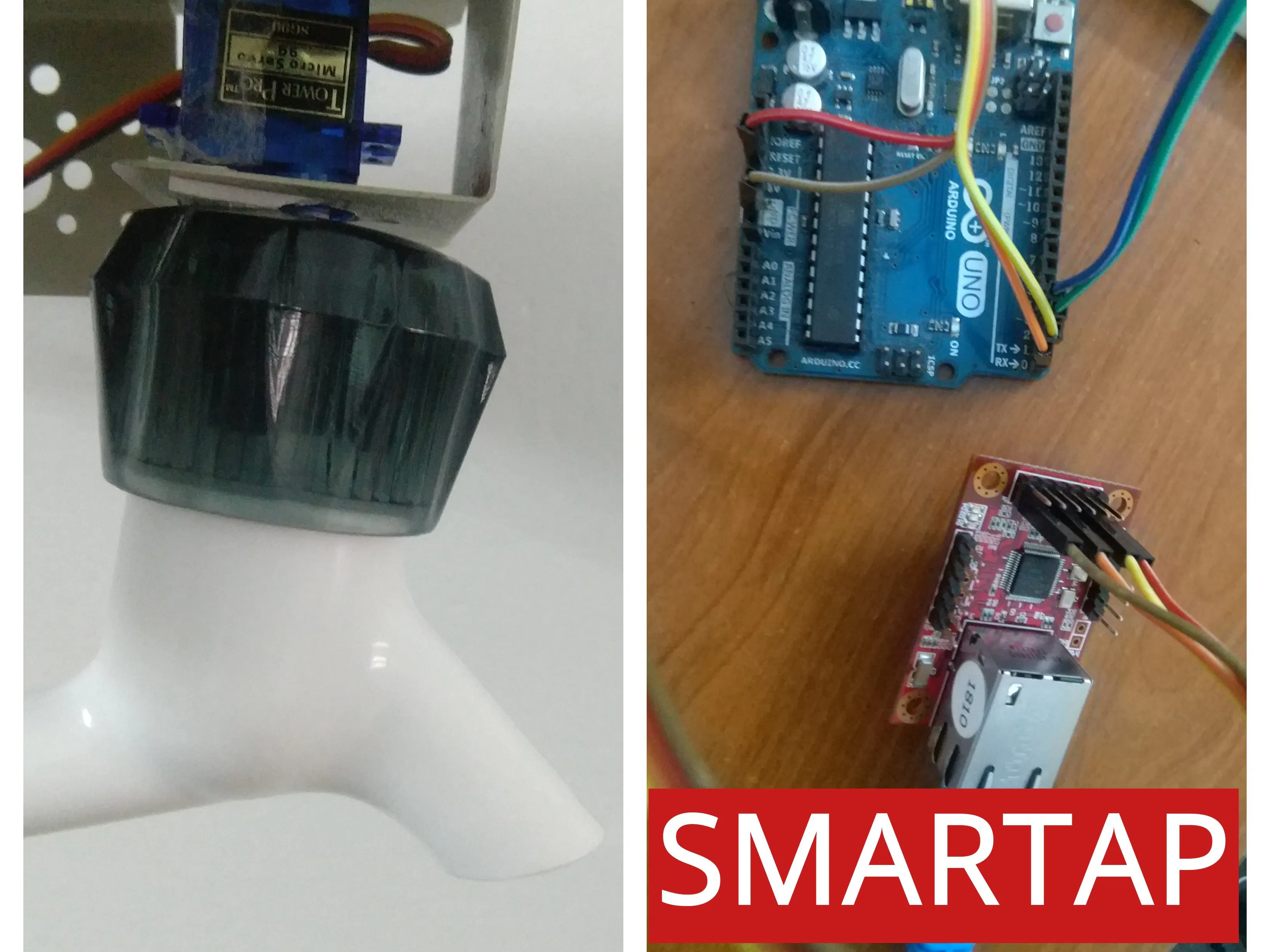## Things used in this project

### Hardware components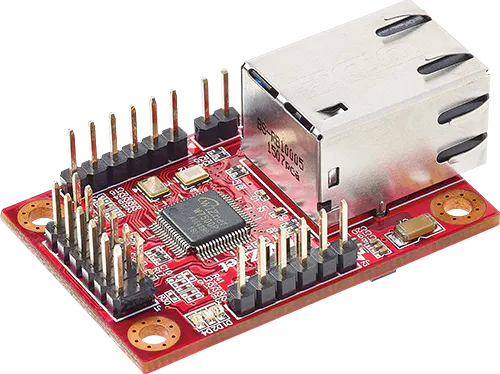WIZnet WIZ750SR
×1Arduino UNO & Genuino UNO
×1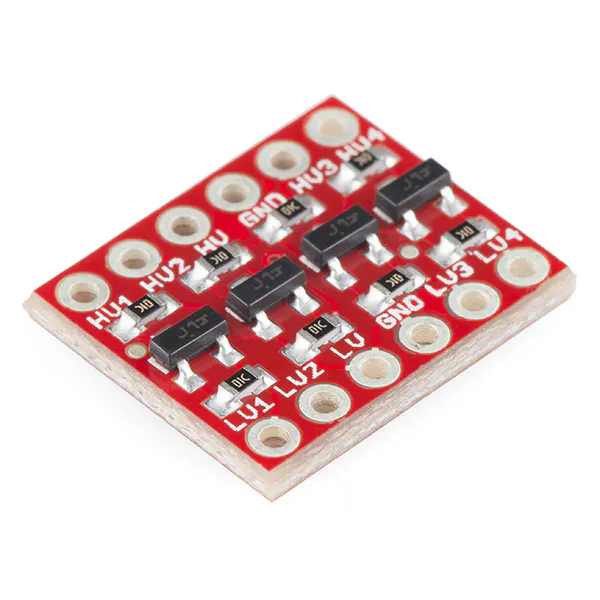SparkFun Logic Level Converter - Bi-Directional
×1
 Water Flow Meter
×1
 3.3V Voltage Regulator(AMS1117-3.3)
×1SparkFun Pushbutton switch 12mm
×1Resistor 10k ohm
×1

### Software apps and online servicesArduino IDE
 Wiznet Configuration tool

## Schematics

### CIrcuit complete

Wiring at the tap end.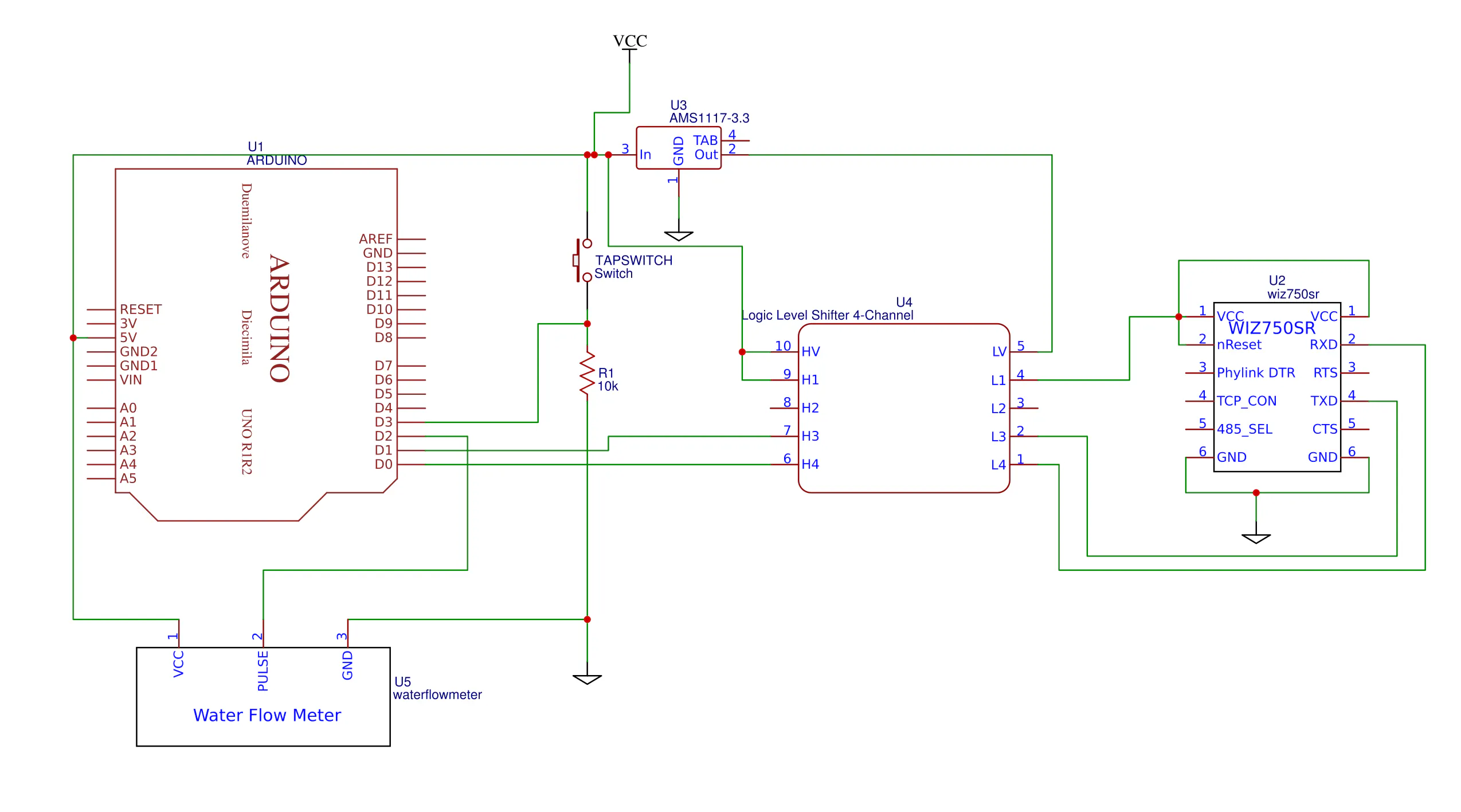### Potential Divider interfacing

Alternate connection for interfacing Wiz750SR with Arduino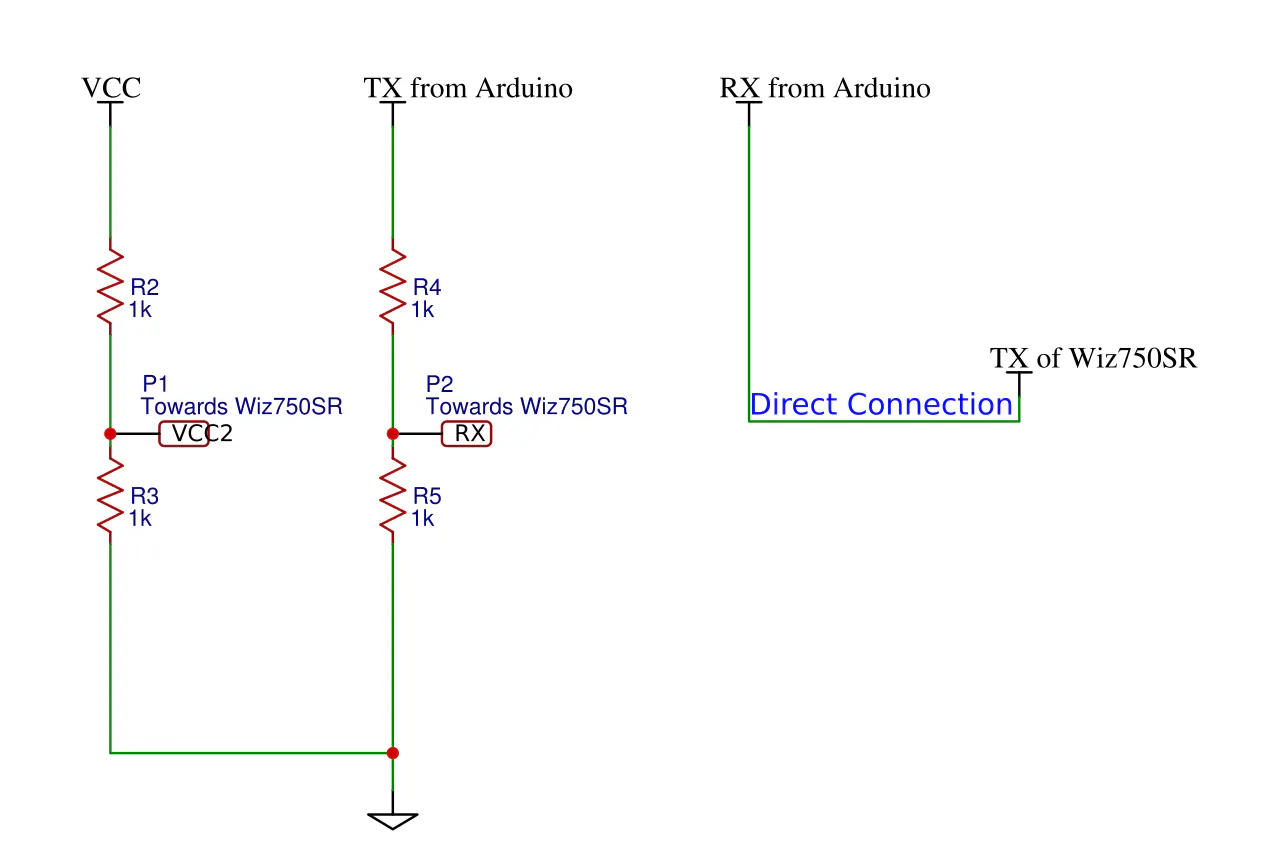## Code

### Complete Arduino code

Arduino
Upload the code directly to arduino
```#include<Servo.h>
#include <avr/pgmspace.h>

#define onswitch 3
#define flowinterrupt 0
#define flowsensor 2

Servo tapservo;

int tapcontrol,duration;
volatile byte tapbutton=LOW;

float calibration=4.5;
volatile byte flowpulse;  //Pulse from flowmeter
float flowRate;
unsigned int flowMilliLitres;
unsigned long totalMilliLitres,threshold;

unsigned long oldTime;

const char PROGMEM webpage1[]="<html>"
" <h2>SMARTAP DATA</h2>"
"  <style>"
"  table, td "
"  {    border: 1px solid black;}"
"  table"
"  {width:100%;}"
"  button"
"  {width: 100%;}"
"  </style>"
"<body>"
"<form action=\"changedata\" method=\"get\" enctype=\"multipart/form-data\" name=\"threshold\" id=\"threshold\" target=\"_self\">"
"<table>"
"  <tr>"
"    <th>Tap state</th>"
"    <td>";
const char PROGMEM webpage2[]="<select>"
"    <option value=\"ON\">ON</option>"
"    <option value=\"OFF\">OFF</option>"
"    </select> "
"  </td>   "
"  </tr>"
"  <tr>"
"    <th>Consumption rate</th>"
"    <td>";
const char PROGMEM webpage3[]="</td>"
"    </tr>"
"    <tr>"
"    <th>Total consumption</th>"
"    <td>";
const char PROGMEM webpage4[]="</td>"
" </tr>"
"    <tr>"
"    <th>Consumption threshold</th>"
"    <td><input type=\"text\" name=\"consthresh\" value='";
const char PROGMEM webpage5[]="' size=\"4\" maxlength=\"3\" />&nbsp;</td></td>"
"    </tr>"
"    <tr>"
"    <th></th>"
"      <td><button type=\"submit\" form=\"threshold\" value=\"Save\">Submit</button></td>"
"    </tr>"
"</table>"
"</form>"
"</body>"
"</html>";

void setup()
{
Serial.begin(115200);
duration=5000;
tapcontrol=250;
tapbutton=false;
pinMode(onswitch, INPUT_PULLUP);
tapservo.attach(9);
threshold=500;    //500ml threshold

//waterflow init
pinMode(flowsensor, INPUT);   //Initialise flow sensor
digitalWrite(flowsensor, HIGH);
flowpulse        = 0;
flowRate          = 0.0;
flowMilliLitres   = 0;
totalMilliLitres  = 0;
oldTime           = 0;
attachInterrupt(flowinterrupt, pulseCounter, FALLING);
attachInterrupt(onswitch, tapclick, FALLING);

}
void loop()
{
serialpeek();    //check if any data is at serial port
}

void serialpeek()
{
int i=0;
char c;
char serialdata;
String datastring="";
if (Serial.available())
{
while (i<100 && Serial.available())
{
serialdata[i]=c;
i++;
}
serialdata[i]=0;
datastring=String(serialdata);
Serial.flush();

if (datastring.indexOf("/ ")>0) { mainPage();}
if (datastring.indexOf("threshold")>0) {updateval(serialdata);}

Serial.flush();
}
}

void mainPage()
{
int i;
byte serbuffer;
for(i=0;i<strlen_P(webpage1);i++)
{
Serial.print(serbuffer);
}
Serial.print(tapbutton);
for(i=0;i<strlen_P(webpage2);i++)
{
Serial.print(serbuffer);
}
Serial.print(flowRate);
for(i=0;i<strlen_P(webpage3);i++)
{
Serial.print(serbuffer);
}
Serial.print(totalMilliLitres);
for(i=0;i<strlen_P(webpage4);i++)
{
Serial.print(serbuffer);
}
Serial.print(threshold);
for(i=0;i<strlen_P(webpage5);i++)
{
Serial.print(serbuffer);
}
}

void updateval(String serialdata)
{
int a,b,x,y,temp=0;
String buffertext,parameter;
x=serialdata.indexOf("?");
y=serialdata.length();
String pagetext = serialdata.substring(x+1,y);
for(int i=0;i<2;i++)
{
x=temp;
y=pagetext.indexOf("&",temp);
buffertext=pagetext.substring(x,y);
temp=x+1;
a=buffertext.indexOf("=");
b=buffertext.length();
parameter[i]=buffertext.substring(a+1,b);
}
if(parameter=="ON")tapclick();
threshold=parameter.toInt();
}

void tapclick()
{
if( totalMilliLitres<= threshold)
{
tapfunc();
}
}

void pulseCounter()
{
flowpulse++;
if((millis() - oldTime) > 1000)
{
detachInterrupt(flowinterrupt);
flowRate = ((1000.0 / (millis() - oldTime)) * flowpulse) / calibration;
oldTime = millis();
flowMilliLitres = (flowRate / 60) * 1000;
totalMilliLitres += flowMilliLitres;
flowpulse = 0;
attachInterrupt(flowinterrupt, pulseCounter, FALLING);
}
}

void tapfunc()
{
tapcontrol=0;
{
tapcontrol+=10;
tapservo.write(tapcontrol);
delay(50);
}
delay(duration);
for(int i=tapcontrol;i>0;i--)   //loop to close the tap with fading effect
{
tapservo.write(i);
delay(30);
}
}
```

### Webpage mainpage

HTML
This code is broken down in the main code
```<html>
<h2>SMARTAP DATA</h2>
<style>
table, td
{    border: 1px solid black;}
table
{width:100%;}
button
{width: 100%;}
</style>
<body>
<form action=\"changedata\" method=\"get\" enctype=\"multipart/form-data\" name=\"threshold\" id=\"threshold\" target=\"_self\">
<table>
<tr>
<th>Tap state</th>
<td>
<select>
<option value="ON">ON</option>
<option value="OFF">OFF</option>
</select>
</td>
</tr>
<tr>
<th>Consumption rate</th>
<td>#</td>
</tr>
<tr>
<th>Total consumption</th>
<td>#</td>
</tr>
<tr>
<th>Consumption threshold</th>
<td><input type=\"text\" name=\"consthresh\" value='0' size=\"4\" maxlength=\"3\" />&nbsp;</td></td>
</tr>
<tr>
<th></th>
<td><button type="submit" form="threshold" value="Save">Submit</button></td>
</tr>
</table>
</form>
</body>
</html>
```

## Credits

### Ferdinand Thomas

1 project • 0 followers